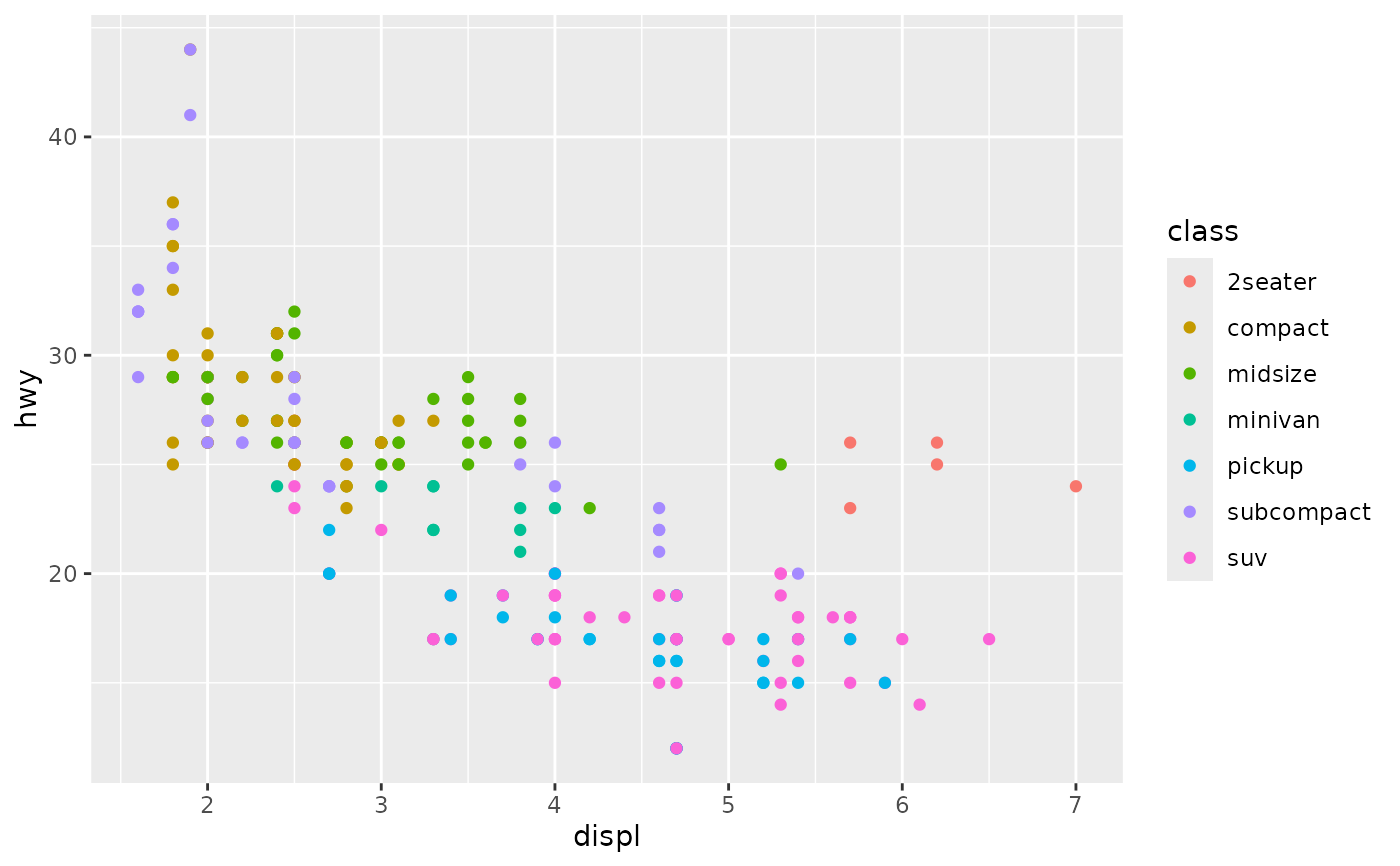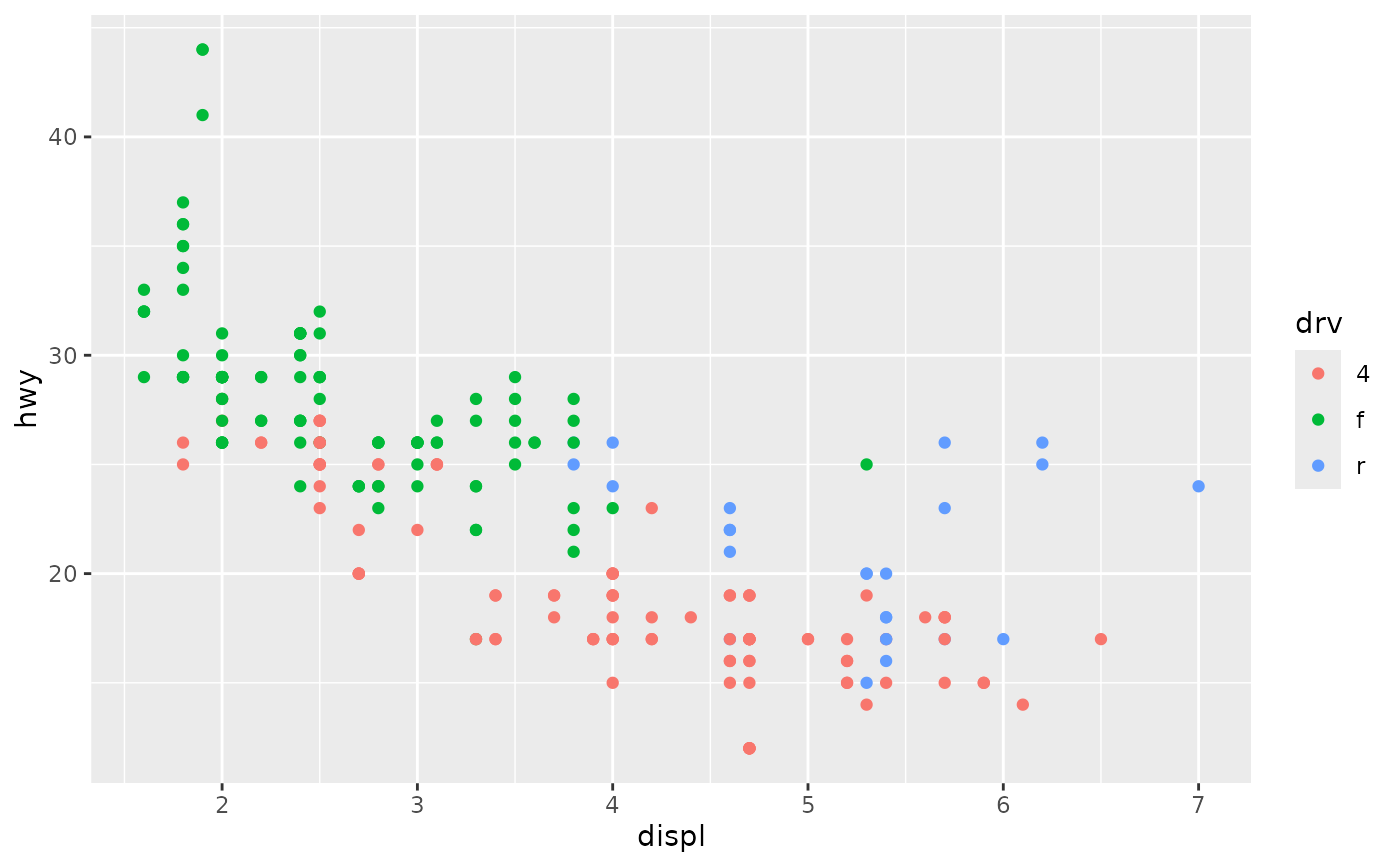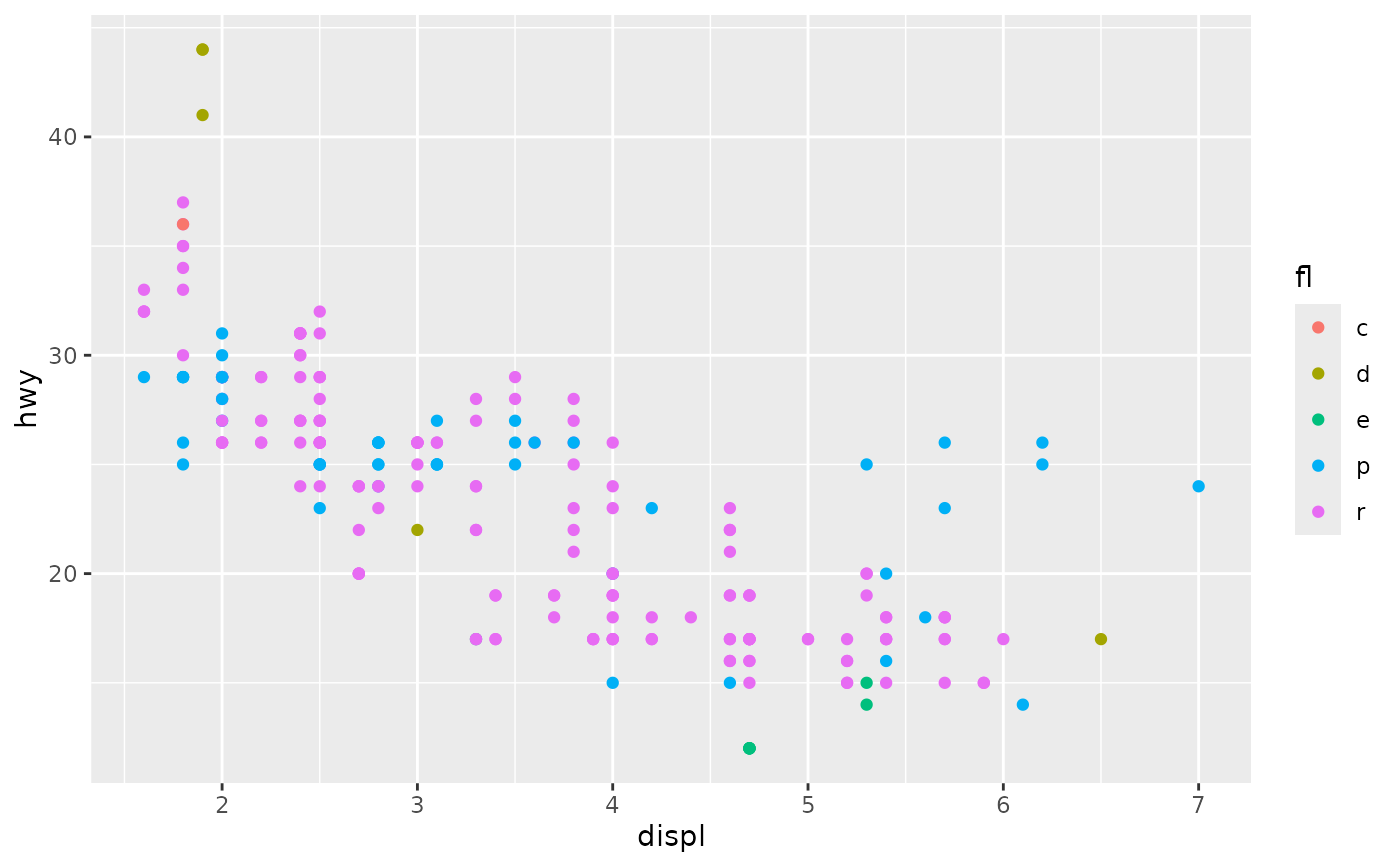Generally, you do not need to print or plot a ggplot2 plot explicitly: the default top-level print method will do it for you. You will, however, need to call print() explicitly if you want to draw a plot inside a function or for loop.

## Usage

# S3 method for ggplot
print(x, newpage = is.null(vp), vp = NULL, ...)

# S3 method for ggplot
plot(x, newpage = is.null(vp), vp = NULL, ...)

## Arguments

x

plot to display

newpage

draw new (empty) page first?

vp

viewport to draw plot in

...

other arguments not used by this method

## Value

Invisibly returns the result of ggplot_build(), which is a list with components that contain the plot itself, the data, information about the scales, panels etc.

## Examples

colours <- list(~class, ~drv, ~fl)

# Doesn't seem to do anything!
for (colour in colours) {
ggplot(mpg, aes_(~ displ, ~ hwy, colour = colour)) +
geom_point()
}

# Works when we explicitly print the plots
for (colour in colours) {
print(ggplot(mpg, aes_(~ displ, ~ hwy, colour = colour)) +
geom_point())
}